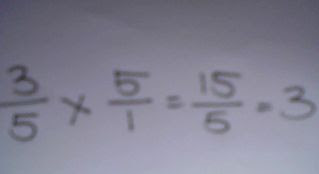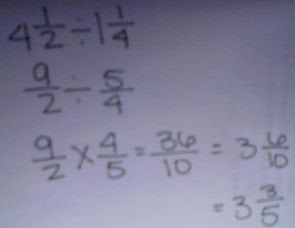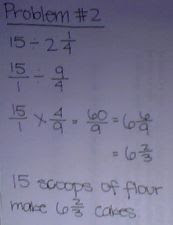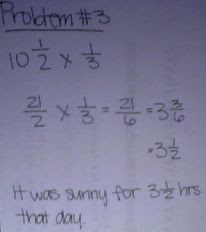### Krissia's Last Fraction Post

Monday, May 11, 2009

1. Find the common denominator.
2. Add the numerator to numerator.
3. Add the denominator to denominator.
4. If it can be simplified, simplify it.Subtracting Fraction

1. Find the common denominator.
2. Subtract the numerator to numerator.

3. Subtract the denominator to denominator.

4. If it can be simplified, simplify it.1. Convert mixed fractions into improper fractions

2. Find the common denominator.

3. Add the numerator to numerator.

4. Add the denominator to denominator.

5. If it can be simplified, simplify it.Multiplying Fractions

1. Multiply the numerator to numerator.

2. Multiply the denominator to denominator.

3. If it can be simplified, simplify it.Multiply Mixed Fractions

1. Convert mixed fractions into improper fractions.
2. Multiply the numerator to numerator.

3. Multiply the denominator to denominator.
4. If the answer is improper, convert improper fractions into mixed fractions.

5. If it can be simplified, simplify it.Dividing Fractions

1. Find the reciprocal of the fraction (flip one of the fraction).
2. Change dividing into multiplying.

3. Multiply the numerator to numerator.
4. Multiply the denominator to denominator.

5. If it can be simplified, simplify it.Dividing Mixed Fractions

1. Convert mixed fractions into improper fractions.
2. Find the reciprocal of the fraction (flip one of the fraction).

3. Change dividing into multiplying.
4. Multiply the numerator to numerator.

5. Multiply the denominator to denominator.
6. If it can be simplified, simplify it.Problem #2Problem #3